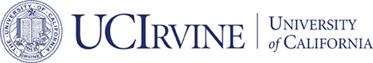# Math 226A Fall 2013## Math 266A Computational PDE

### Meeting Information

• Room: RH 440R
• Day & time: M W F 10 - 11 am.

### Project and Homework

• Homework 1: Exercises in Lecture Notes for Finite Difference Methods. Due date: Oct 10, 2013.
• Project 1: Finite Difference Method. Due date: Oct 18.

Use publish to summarize your project. A quick introduction on the publish function in matlab can be found in Publish MATLAB Code and Publishing Markup.

### Syllabus

In Math 226 A, we shall focus on numerical solutions for the elliptic equations. Parabolic and hyperbolic equations will be discussed in 226 B and C, respectively. More precisely, we plan to cover the following topics:

• Finite element methods for linear elliptic equations
• Finite difference and finite volume methods
• Sobolev spaces and elliptic equations
• Fast solvers: Conjugate Gradient method and multigrid method
• Nonlinear elliptic equations
• Fast multiple methods

### References

Lecture Notes will be distributed in class. But the following books are good references for reading:

• Gilbert Strang. Computational Science and Engineering, Wellesley-Cambridge Press, 2007. Watch viedos in iTunes U.
• S. Larsson and V. Thomee, Partial Differential Equations with Numerical Methods, Springer, 2003.
• S.C. Brenner and L.R. Scott. The mathematical theory of finite element methods, vol. 15 of Texts in Applied Mathematics, Springer-Verlag, New York, second edition, 2002.